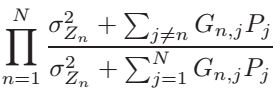# How to write my Objective for Geometric Prog

Hi,
I am solving a GP with the objective which a production of the terms as

“(b_{j} + \sum_{i \neq j}A{i,j}x_{i})\prod_{i}(x_{i}/a_{i})^{\alpha_{i}}”

for i = 1,…,N

N might be very large thus a choice is to use prod(.) operator in MATLAB but it doesn’t work.

Can Anyone help me?

To be more clear my objective is as follows where I have approximated the posynomial denominator by a monomial in order to make the signomial prog. a GP and thus solvable.